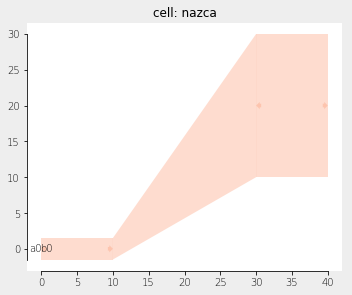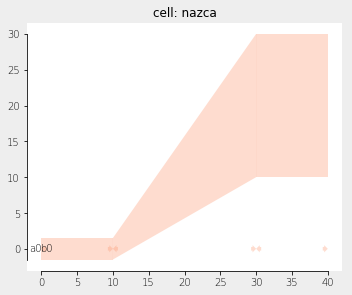Home Forums Nazca Connecting two dissimilar ribbons Reply To: Connecting two dissimilar ribbons

#5714

Dear mbjvrijn,

The shape in your second code samplecan be reproduced using the relations for a and b in my previous post. In the code below I took y1 and y2 from the output of your code. The centre line of the interconnect lies at y=0.

``````import nazca as nd

# get (a, b) for the left and right edge:
def get_edge(y1, y2):
w1 = 1.0 # w1 and w2 can be chosen arbitrary, but not the same
w2 = 2.0
a = (y1 - y2) / (w1 - w2)
b = (y2*w1 - y1*w2) / (w1 - w2)
return a, b

al, bl = get_edge(y1=1.5, y2=30)
ar, br = get_edge(y1=-1.5, y2=10)

# The actual xsection definition: